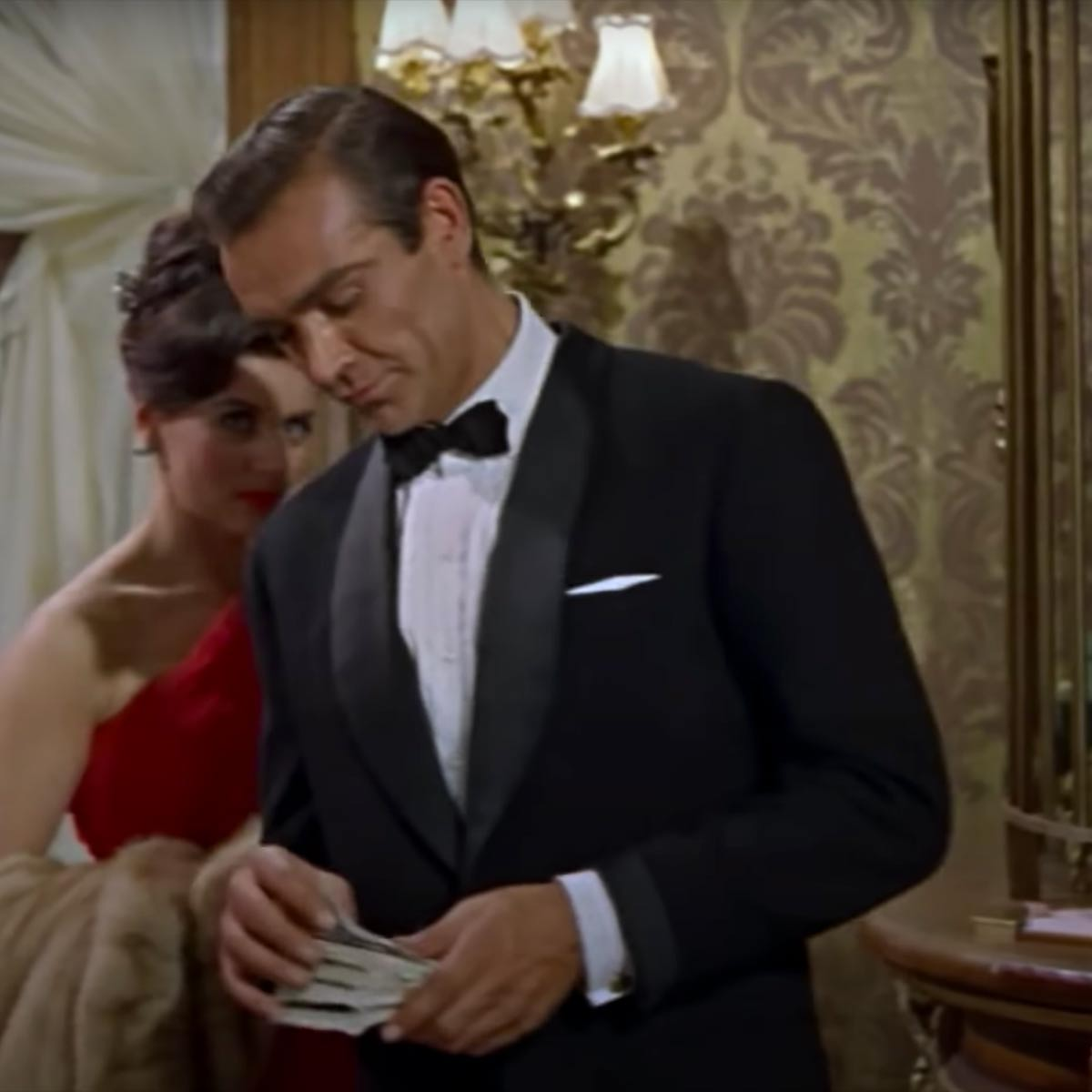# James Bond (Theme)

10 September 2020
4
00:40
-5
• ##### TEMPO
120
0
(0)

u||i||
I||i||
u||i||
I||i||
p aaa a|p p p
p sss s|a a a
p aaa a|p p p
p sss s|a a a
p aaa a|p p p
p sss s|a a p H [hf]||
[sf] [da] [sf]

p aaa a|p p p
p sss s|a a a
p aaa a|p p p
p sss s|a a a
p aaa a|p p p
p sss s|a a p H [hf]||
[sf] [da] [sf]

Rate This Music Sheet:

Average rating 0 / 5. Vote count: 0

No votes so far! Be the first to rate this music sheet.

Thank you for rating this song!

If you have any specific feedback about how to improve this music sheet, please submit this in the box below.

James Bond (Theme) is a song by Monty Norman. Use your computer keyboard to play James Bond (Theme) music sheet on Virtual Piano. This is an Easy song and requires practice. The recommended time to play this music sheet is 00:40, as verified by Virtual Piano legend, Mark Chaimbers. The song James Bond (Theme) is classified in the genre of Songs From Movies on Virtual Piano. You can also find other similar songs using James Bond, Theme Song.

## Related

•l k l z|l z c|x z l|| j|k|l|z|l k l z|l|l|k l z|l|x| c x|z|l|| l|k|j|g|h|j|l|k j|k|ll k l h g f s| f f g h h| g f|s|d|| d d f g g||d|a|s|| s|j|h|g|f|s|d| f f|| g|f|g| g|| g g g f| d|s d|h|| g h l|f|d|s| g||f||d|| s|s|f|| gfdh|h|h h h h s|s|| h h h h h h h h|s s|d f| d d s d|s f| g|h|j||| k|j|H|| j| kl k l z|h h z|l k l|| l k l z|l z x||| l k l z|h h z|l k l|| x x x z|l k jl k l z|h|z|l k l|| h l k l z|v c x x||| x x x z|l|z|x c x|| h x x x z|l k jl|k|l|k|l|k|j|h|h j h j| h j h j| j|k| l|k|l|k|l|k|l|z|x x x z|l k j
Level: 2
Length: 02:02
Easy

#### Goose House

•u p s [tp] p s p [ya] p i o [ue] |
u p s [tp] p s p [ew] p u Y [yw] |
y i O [rw] |
y i O [pw] |
e t o [iw] u o i [iw] u u W [ew]

Level: 4
Length: 00:30
Easy

#### Nino Rota

•u o s f f f d g f s | u u o s s s a d s | s s s s a d s o | u o o o i p o | s s s s a d s o | u o o o i p o | p s s p o s o s | d d d s f d s | p s s p o s o s | d d d s f d s | f s | f d s p f d s d | f d s p o f d s p o f d s f | f d s p p s d d | f d s d d f f s | f d s p o f d s p o f d s f
Level: 2
Length: 01:25
Easy